﻿ 一类具有时滞效应与脉冲控制的捕食–食饵系统的动力学研究 Dynamic Analysis of a Predator-Prey System with Time Delay and Pulse Control

Vol.07 No.08(2018), Article ID:26534,13 pages
10.12677/AAM.2018.78116

Dynamic Analysis of a Predator-Prey System with Time Delay and Pulse Control

Jing Yang

College of Mathematics Physics and Electronic Information Engineering, Wenzhou University, Wenzhou Zhejiang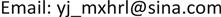Received: Aug. 1st, 2018; accepted: Aug. 15th, 2018; published: Aug. 22nd, 2018ABSTRACT

Based on theory of ecological dynamics and ecological control modeling idea, a predator-prey dynamic system with time-delay and pulse control is constructed. The sufficient criterion of local asymptotic stability and global attraction for the semi-trivial periodic solution of the system is established. The permanence of the system is proved. Further, the dynamic behavior of the model was simulated numerically, which verifies the validity and feasibility of theoretical analysis.

Keywords:Pulse Control, Time Delay, Stability, Global Attraction, Persistence1. 引言

$\left\{\begin{array}{l}{\stackrel{˙}{N}}_{i}\left(t\right)=B\left(t\right)-{D}_{i}\left(t\right)-W\left(t\right)\\ {\stackrel{˙}{N}}_{m}\left(t\right)=\alpha W\left(t\right)-{D}_{m}\left(t\right)\end{array}$ (1)

$\left\{\begin{array}{l}\stackrel{˙}{x}\left(t\right)=\beta y\left(t\right)-rx\left(t\right)-\beta {\text{e}}^{-r\tau }y\left(t-\tau \right)\\ \stackrel{˙}{y}\left(t\right)=\beta {\text{e}}^{-r\tau }y\left(t-\tau \right)-{\eta }_{2}{y}^{2}\left(t\right)\end{array}$ (2)

$\stackrel{˙}{N}\left(t\right)=N\left(t\right)\left(r-aN\left(t\right)\right)$ (3)

$\left\{\begin{array}{l}\stackrel{˙}{x}\left(t\right)=\left[r-a\left(x\left(t\right)+y\left(t\right)\right)\right]x\left(t\right)-r{\text{e}}^{-{d}_{1}\tau }y\left(t-\tau \right)-{d}_{1}x\left(t\right)\\ \stackrel{˙}{y}\left(t\right)=r{\text{e}}^{-{d}_{1}\tau }y\left(t-\tau \right)+\left[r-{d}_{2}-a\left(x\left(t\right)+y\left(t\right)\right)\right]y\left(t\right)-by\left(t\right)z\left(t\right)\\ \stackrel{˙}{z}\left(t\right)=kby\left(t\right)z\left(t\right)-{d}_{3}z\left(t\right)\end{array}$ (4)

$\left\{\begin{array}{l}\begin{array}{l}\stackrel{˙}{x}\left(t\right)=\left[r-a\left(x\left(t\right)+y\left(t\right)\right)\right]x\left(t\right)-r{\text{e}}^{-{d}_{1}\tau }y\left(t-\tau \right)-{d}_{1}x\left(t\right)\\ \stackrel{˙}{y}\left(t\right)=r{\text{e}}^{-{d}_{1}\tau }y\left(t-\tau \right)+\left[r-{d}_{2}-a\left(x\left(t\right)+y\left(t\right)\right)\right]y\left(t\right)-by\left(t\right)z\left(t\right)\\ \stackrel{˙}{z}\left(t\right)=kby\left(t\right)z\left(t\right)-{d}_{3}z\left(t\right)\end{array}\right\}t\ne nT\\ \begin{array}{l}\Delta x\left(t\right)=0\\ \Delta y\left(t\right)=0\\ \Delta z\left(t\right)=R\end{array}\right\}t=nT\end{array}$ (5)

$\left\{\begin{array}{l}\left({\varphi }_{1},{\varphi }_{2},\psi \right)\in \left(\left[-\tau ,0\right],{R}_{3}^{+}\right),\\ x\left(t\right)={\varphi }_{1}\left(t\right),y\left(t\right)={\varphi }_{2}\left(t\right),z\left(t\right)=\psi \left(t\right),\\ {R}_{3}^{+}=\left\{x\in {R}^{3}:x\ge 0\right\}.\end{array}$ (6)

$D=\left\{\left(x\left(t\right),y\left(t\right),z\left(t\right)\right):x\left(t\right)>0,y\left(t\right)>0,z\left(t\right)>0\right\}$ (7)

2. 定理

2) V对于X满足局部的Lipschitz条件。

$\left\{\begin{array}{l}\stackrel{˙}{w}\left(t\right)\le p\left(t\right)w\left(t\right)+q\left(t\right),\text{\hspace{0.17em}}\text{\hspace{0.17em}}t\ne {\tau }_{i},\text{\hspace{0.17em}}\text{\hspace{0.17em}}t>0\\ w\left({t}^{+}\right)\le {p}_{i}w\left(t\right)+{q}_{i},\text{\hspace{0.17em}}\text{\hspace{0.17em}}t=n{\tau }_{i}>0\\ u\left({0}^{+}\right)\le {w}_{0},\end{array}$ (8)

$\begin{array}{l}w\left(t\right)\le w\left(0\right)\frac{\Pi }{0<{\tau }_{i}

$\left\{\begin{array}{l}\stackrel{˙}{h}\left(t\right)=-{d}_{3}h\left(t\right)\text{\hspace{0.17em}}\text{\hspace{0.17em}}\text{\hspace{0.17em}}\text{\hspace{0.17em}}\text{\hspace{0.17em}}t\ne nT\\ h\left({t}^{+}\right)=h\left(t\right)+R\text{\hspace{0.17em}}\text{\hspace{0.17em}}\text{\hspace{0.17em}}\text{\hspace{0.17em}}t=nT\\ h\left({0}^{+}\right)={h}_{0}\end{array}$ (9)

${h}^{*}\left(t\right)=\frac{R{e}^{-{d}_{3}\left(t-nT\right)}}{1-{\text{e}}^{-{d}_{3}T}},\text{\hspace{0.17em}}\text{\hspace{0.17em}}\text{\hspace{0.17em}}\text{\hspace{0.17em}}t\in \left(nT,\left(n+1\right)T\right]$ .

$\frac{\text{d}u}{\text{d}t}=au\left(t-\tau \right)-b\left(t\right)u\left(t\right)$ , (10)

1) 若 $a>b$ ，则 $\underset{t\to \infty }{\mathrm{lim}}u\left(t\right)=+\infty$

2) 若 $a\le b$ ，则 $\underset{t\to \infty }{\mathrm{lim}}u\left(t\right)=0$

$\frac{\text{d}u}{\text{d}t}=au\left(t-\tau \right)-bu\left(t\right)-c{u}^{2}\left(t\right)$ , (11)

1) 若 $a>b$ ，则 $\underset{t\to \infty }{\mathrm{lim}}u\left(t\right)=\frac{a-b}{c}$

2) 若 $a\le b$ ，则 $\underset{t\to \infty }{\mathrm{lim}}u\left(t\right)=0$

3. 理论分析

3.1. 系统(5)任意解的有界性

$t\ne nT$ 时，

$\begin{array}{l}{D}^{+}V\left(t\right)=k\left[r-a\left(x\left(t\right)+y\left(t\right)\right)\right]x\left(t\right)-kr{\text{e}}^{-{d}_{1}\tau }y\left(t-\tau \right)-k{d}_{1}x\left(t\right)+kr{\text{e}}^{-{d}_{1}\tau }y\left(t-\tau \right)\\ \text{\hspace{0.17em}}\text{\hspace{0.17em}}\text{\hspace{0.17em}}\text{\hspace{0.17em}}\text{\hspace{0.17em}}\text{\hspace{0.17em}}\text{\hspace{0.17em}}\text{\hspace{0.17em}}\text{\hspace{0.17em}}\text{\hspace{0.17em}}\text{\hspace{0.17em}}\text{\hspace{0.17em}}\text{\hspace{0.17em}}\text{\hspace{0.17em}}+k\left[r-{d}_{2}-a\left(x\left(t\right)+y\left(t\right)\right)\right]y\left(t\right)-kby\left(t\right)z\left(t\right)+kby\left(t\right)z\left(t\right)-{d}_{3}z\left(t\right)\\ \text{\hspace{0.17em}}\text{\hspace{0.17em}}\text{\hspace{0.17em}}\text{\hspace{0.17em}}\text{\hspace{0.17em}}\text{\hspace{0.17em}}\text{\hspace{0.17em}}\text{\hspace{0.17em}}\text{\hspace{0.17em}}\text{\hspace{0.17em}}\text{\hspace{0.17em}}=k\left[-a{x}^{2}\left(t\right)+\left(r-{d}_{1}+{d}_{3}\right)x\left(t\right)\right]-2kax\left(t\right)y\left(t\right)\\ \text{\hspace{0.17em}}\text{\hspace{0.17em}}\text{\hspace{0.17em}}\text{\hspace{0.17em}}\text{\hspace{0.17em}}\text{\hspace{0.17em}}\text{\hspace{0.17em}}\text{\hspace{0.17em}}\text{\hspace{0.17em}}\text{\hspace{0.17em}}\text{\hspace{0.17em}}\text{\hspace{0.17em}}\text{\hspace{0.17em}}\text{\hspace{0.17em}}+k\left[-a{y}^{2}\left(t\right)+\left(r-{d}_{2}+{d}_{3}\right)y\left(t\right)\right]-{d}_{3}V\left(t\right)\end{array}$

${D}^{+}V\left(t\right)+{d}_{3}V\left(t\right)\le \frac{k{\left(r-{d}_{1}+{d}_{3}\right)}^{2}}{4a}+\frac{k{\left(r-{d}_{2}+{d}_{3}\right)}^{2}}{4a}=\frac{k\left[{\left(r-{d}_{1}+{d}_{3}\right)}^{2}+{\left(r-{d}_{2}+{d}_{3}\right)}^{2}\right]}{4a}={M}_{0}$

$\left\{\begin{array}{l}{D}^{+}V\left(t\right)\le -{d}_{3}V\left(t\right)+{M}_{0}\text{\hspace{0.17em}}\text{\hspace{0.17em}}\text{\hspace{0.17em}}\text{\hspace{0.17em}}t\ne nT\\ V\left({t}^{+}\right)=V\left(t\right)+R\text{\hspace{0.17em}}\text{\hspace{0.17em}}\text{\hspace{0.17em}}\text{\hspace{0.17em}}\text{\hspace{0.17em}}t=nT\end{array}$ (12)

$\begin{array}{l}V\left(t\right)\le \left[V\left({0}^{+}\right)-\frac{{M}_{0}}{{d}_{3}}\right]{\text{e}}^{-{d}_{3}t}+\frac{R{e}^{-{d}_{3}\left(t-nT\right)}\left(1-{\text{e}}^{-{d}_{3}nT}\right)}{1-{\text{e}}^{-{d}_{3}T}}+\frac{{M}_{0}}{{d}_{3}}\text{\hspace{0.17em}}\text{\hspace{0.17em}}\text{\hspace{0.17em}}\text{\hspace{0.17em}}t\in \left(nT,\left(n+1\right)T\right]\\ \text{\hspace{0.17em}}\text{\hspace{0.17em}}\text{\hspace{0.17em}}\text{\hspace{0.17em}}\text{\hspace{0.17em}}\text{\hspace{0.17em}}\text{\hspace{0.17em}}\le \frac{R{e}^{{d}_{3}T}}{1-{\text{e}}^{-{d}_{3}T}}+\frac{{M}_{0}}{{d}_{3}}\triangleq M\text{\hspace{0.17em}}\text{\hspace{0.17em}}\text{\hspace{0.17em}}\text{\hspace{0.17em}}\text{\hspace{0.17em}}\left(t\to \infty \right)\end{array}$ (13)

3.2. 系统(5)半平凡周期解的局部渐近稳定性

$\left\{\begin{array}{l}\begin{array}{l}\stackrel{˙}{H}\left(t\right)=\left(r-{d}_{1}\right)H\left(t\right)-r{\text{e}}^{-{d}_{1}\tau }P\left(t\right)\\ \stackrel{˙}{P}\left(t\right)=\left[r-{d}_{2}-b{z}^{*}\left(t\right)+r{\text{e}}^{-{d}_{1}\tau }\right]P\left(t\right)\\ \stackrel{˙}{W}\left(t\right)=kb{z}^{*}\left(t\right)P\left(t\right)-{d}_{3}W\left(t\right)\end{array}\right\}t\ne nT\\ \begin{array}{l}H\left({t}^{+}\right)=H\left(t\right)\\ P\left({t}^{+}\right)=P\left(t\right)\\ W\left({t}^{+}\right)=W\left(t\right)+R\end{array}\right\}t=nT\end{array}$ (14)

$\frac{\text{d}\varphi \left(t\right)}{\text{d}t}=A\left(t\right)\varphi \left(t\right)$ (15)

$t=nT$ 时， $\left[\begin{array}{c}H\left(n{T}^{+}\right)\\ P\left(n{T}^{+}\right)\\ W\left(n{T}^{+}\right)\end{array}\right]=\left[\begin{array}{ccc}1& 0& 0\\ 0& 1& 0\\ 0& 0& 1\end{array}\right]\left[\begin{array}{c}H\left(nT\right)\\ P\left(nT\right)\\ W\left(nT\right)\end{array}\right]$

$\Phi \left(T\right)=\varphi \left(0\right){\text{e}}^{\underset{0}{\overset{T}{\int }}A\left(s\right)\text{d}s}\triangleq \varphi \left(0\right)\mathrm{exp}\left(\stackrel{˜}{A}\right),\text{\hspace{0.17em}}\stackrel{˜}{A}=\underset{0}{\overset{T}{\int }}A\left(s\right)\text{d}s$ (16)

${\lambda }_{1}={\text{e}}^{\underset{0}{\overset{T}{\int }}\left(r-{d}_{1}\right)\text{d}s},\text{\hspace{0.17em}}{\lambda }_{2}={\text{e}}^{\underset{0}{\overset{T}{\int }}\left[r-{d}_{2}-b{z}^{*}\left(s\right)+r{\text{e}}^{-{d}_{1}\tau }\right]\text{d}s},\text{\hspace{0.17em}}{\lambda }_{3}={\text{e}}^{\underset{0}{\overset{T}{\int }}\left(-{d}_{3}\right)\text{d}s}={\text{e}}^{-{d}_{3}T}<1$ (17)

$z\left(t\right)=\left[{z}_{0}-\frac{R}{1-{\text{e}}^{-{d}_{3}T}}\right]{\text{e}}^{-{d}_{3}t}+{z}^{*}\left(t\right),\text{\hspace{0.17em}}t\in \left(nT,\left(n+1\right)T\right]$ . (18)

3.3. 系统(5)半平凡周期解的全局吸引性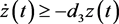(19)(20)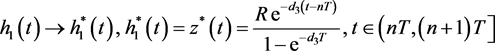. (21)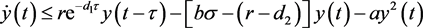(22)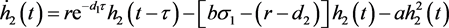(23)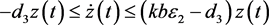(24)(25)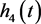是系统(26)的解。(26)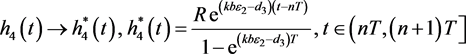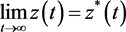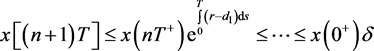(27)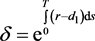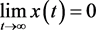3.4. 系统(5)的持久生存性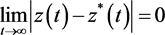，知对任意的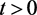，存在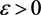，使得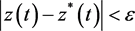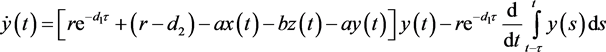(28)(29)

(H1)：假设对上面的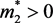，对任意的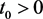，当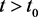时，有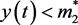不恒成立，则可以假设存在，使得当时有成立。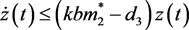(30)(31)(32)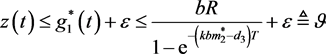(33)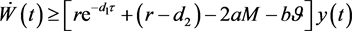(34)(35)

(H2)：根据第一个假设，只需要考虑两种情况，

1)对所有的充分大的t，都有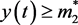成立；

2)当t充分大时，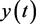关于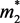左右波动。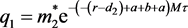(36)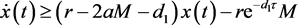(37)

4. 数值模拟

5. 结论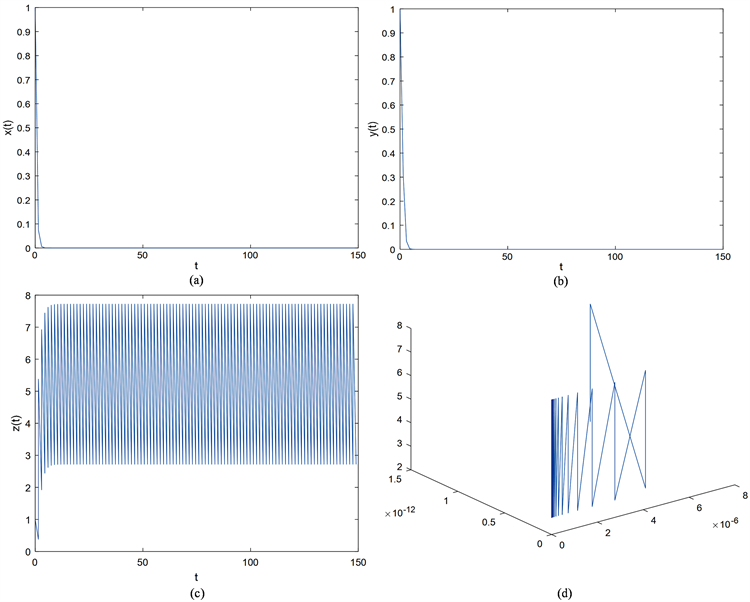Figure 1. Time diagram and phase diagram of system (5) when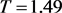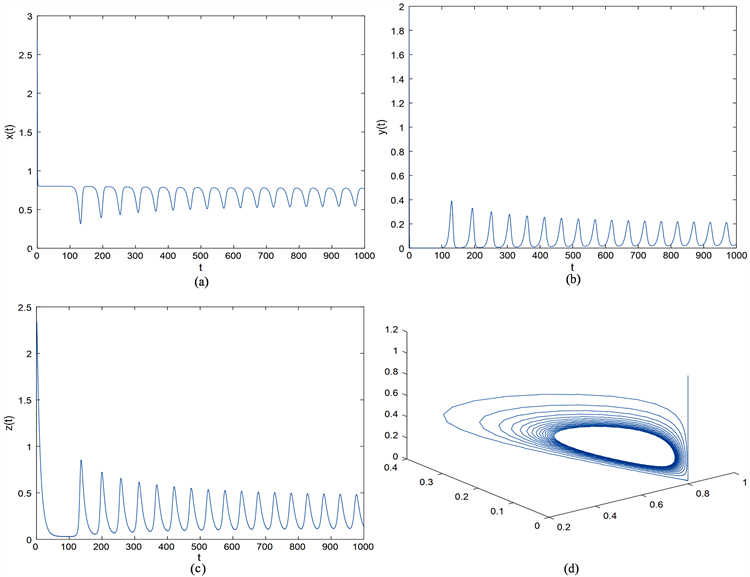Figure 2. Time diagram and phase diagram of system (5) when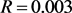Dynamic Analysis of a Predator-Prey System with Time Delay and Pulse Control[J]. 应用数学进展, 2018, 07(08): 987-999. https://doi.org/10.12677/AAM.2018.78116

1. 1. 宋燕, 杜月, 李紫薇. 具有阶段结构及脉冲控制的害虫管理模型[J]. 渤海大学学报(自然科学版), 2017, 38(2): 104-110.

2. 2. Cai, S., Jiao, J. and Li, L. (2015) Dynamics of a Pest Management Predator-Prey Model with Stage Structure and Im-pulsive Stocking. Journal of Applied Mathematics & Computing, 52, 125-138. https://doi.org/10.1007/s12190-015-0933-3

3. 3. Grasman, J., van Herwaarden, O.A., Hemerik, L. and van Lenteren, J.C. (2001) A Two-Component Model of Host-Parasitoid Interactions: Determination of the Size of Inundative Releases of Parasitoids in Biological Pest Control. Mathematical Biosciences, 169, 207-216. https://doi.org/10.1016/S0025-5564(00)00051-1

4. 4. Liu, X. and Chen, L. (2003) Complex Dynamics of Holling Type ii Lotka-Volterra Predator-Prey System with Impulsive Perturbations on the Predator. Chaos Solitons & Fractals, 16, 311-320. https://doi.org/10.1016/S0960-0779(02)00408-3

5. 5. Panetta, J.C. (1996) A Mathematical Model of Periodically Pulsed Che-motherapy: Tumor Recurrence and Metastasis in a Competitive Environment. Bulletin of Mathematical Biology, 58, 425-447. https://doi.org/10.1007/BF02460591

6. 6. Barclay, H.J. (1982) Models for Pest Control Using Predator Release, Habitat Man-agement and Pesticide Release in Combination. Journal of Applied Ecology, 19, 337-348. https://doi.org/10.2307/2403471

7. 7. Lotka, A.J. (1927) Scientific Books: Elements of Physical Biology. Science, 66, 281-282. https://doi.org/10.1126/science.66.1708.281-a

8. 8. Hethcote, H.W., Wang, W., Han, L. and Ma, Z. (2004) A Predator-Prey Model with Infected Prey. Theoretical Population Biology, 66, 259-268. https://doi.org/10.1016/j.tpb.2004.06.010

9. 9. Liu, S. and Beretta, E. (2006) A Stage-Structured Predator-Prey Model of Beddington-Deangelis Type. Siam Journal on Applied Mathematics, 66, 1101-1129. https://doi.org/10.1137/050630003

10. 10. Gourley, S.A. and Lou, Y. (2014) A Mathematical Model for the Spatial Spread and Biocontrol of the Asian Longhorned Beetle. Siam Journal on Applied Mathematics, 74, 2-11. https://doi.org/10.1137/130939304

11. 11. Yu, H., Zhao, M., Wang, Q. and Agarwal, R.P. (2014) A Focus on Long-Run Sustai-nability of an Impulsive Switched Eutrophication Controlling System Based upon the Zeya Reservoir. Journal of the Franklin Institute, 351, 487-499. https://doi.org/10.1016/j.jfranklin.2013.08.025

12. 12. Zhang, Y., Chen, S., Gao, S. and Wei, X. (2017) Stochastic Periodic Solution for a Perturbed Non-Autonomous Predator-Prey Model with Generalized Nonlinear Harvesting and Impulses. Physica A: Statistical Mechanics & Its Applications, 486, 347-366. https://doi.org/10.1016/j.physa.2017.05.058

13. 13. Yu, H., Zhong, S. and Agarwal, R.P. (2011) Mathematics Analysis and Chaos in an Ecological Model with an Impulsive Control Strategy. Communications in Nonlinear Science & Numerical Simulation, 16, 776-786. https://doi.org/10.1016/j.cnsns.2010.04.017

14. 14. Xie, Y., Wang, L., Deng, Q. and Wu, Z. (2017) The Dynamics of an Impulsive Predator-Prey Model with Communicable Disease in the Prey Species Only. Applied Mathematics & Computation, 292, 320-335. https://doi.org/10.1016/j.amc.2016.07.042

15. 15. Wang, Z., Shao, Y., Fang, X. and Ma, X. (2016) The Dynamic Behaviors of One-Predator Two-Prey System with Mutual Interference and Impulsive Control. Mathematics & Computers in Simulation, 132, 68-85. https://doi.org/10.1016/j.matcom.2016.06.007

16. 16. Ding, X. and Jiang, J. (2009) Periodicity in a Generalized Semi-Ratio-Dependent Predator-Prey System with Time Delays and Impulses. Journal of Mathematical Analysis & Applications, 360, 223-234. https://doi.org/10.1016/j.jmaa.2009.06.048

17. 17. Pei, Y., Liu, S., Li, C. and Chen, L. (2009) The Dynamics of an Impulsive Delay Si Model with Variable Coefficients. Applied Mathematical Modelling, 33, 2766-2776. https://doi.org/10.1016/j.apm.2008.08.011

18. 18. Tan, R., Liu, Z. and Cheke, R.A. (2012) Periodicity and Stability in a Sin-gle-Species Model Governed by Impulsive Differential Equation. Applied Mathematical Modelling, 36, 1085-1094. https://doi.org/10.1016/j.apm.2011.07.056

19. 19. Dai, C., Zhao, M. and Chen, L. (2012) Complex Dynamic Behavior of Three-Species Ecological Model with Impulse Perturbations and Seasonal Disturbances. Mathematics & Computers in Simulation, 84, 83-97. https://doi.org/10.1016/j.matcom.2012.09.004

20. 20. 卢肠. 几类捕食-食饵模型的定性分析[J]. 哈尔滨: 哈尔滨工业大学, 2017.

21. 21. Khajanchi, S. and Banerjee, S. (2017) Role of Constant Prey Refuge on Stage Structure Predator-Prey Model with Ratio Dependent Functional Response. Applied Mathematics & Computation, 314, 193-198. https://doi.org/10.1016/j.amc.2017.07.017

22. 22. Jiao, J. and Chen, L. (2008) Global Attractivity of a Stage-Structure Variable Coefficients Predator-Prey System with Time Delay and Impulsive Perturbations on Predators. International Journal of Biomathe-matics, 1, 197-208. https://doi.org/10.1142/S1793524508000163

23. 23. Zhang, H. and Chen, L. (2008) A Model for Two Species with Stage Structure and Feedback Control. International Journal of Biomathematics, 1, 267-286. https://doi.org/10.1142/S1793524508000230

24. 24. Shao, Y. and Ma, X. (2017) Analysis of a Predator-Prey System with Bed-dington-Type Functional Response and Stage-Structure of Prey. International Journal of Dynamical Systems & Differential Equations, 7, 51. https://doi.org/10.1504/IJDSDE.2017.083727

25. 25. Sailer, R.I. (1975) Book Review: Biological Control by Natural Ene-mies.

26. 26. Chen, F., Xie, X. and Li, Z. (2012) Partial Survival and Extinction of a Delayed Predator-Prey Model with Stage Structure. Applied Mathematics & Computation, 219, 4157-4162. https://doi.org/10.1016/j.amc.2012.10.055

27. 27. Aiello, W.G. and Freedman, H.I. (1990) A Time-Delay Model of Single-Species Growth with Stage Structure. Mathematical Biosciences, 101, 139. https://doi.org/10.1016/0025-5564(90)90019-U

28. 28. Pei, Y., Li, C. and Fan, S. (2013) A Mathematical Model of a Three Species Prey-Predator System with Impulsive Control and Holling Functional Response. Applied Mathematics and Computation, 219, 10945-10955. https://doi.org/10.1016/j.amc.2013.05.012

29. 29. Pang, G., Wang, F. and Chen, L. (2009) Extinction and Permanence in Delayed Stage-Structure Predator-Prey System with Impulsive Effects. Chaos Solitons & Fractals, 39, 2216-2224. https://doi.org/10.1016/j.chaos.2007.06.071

30. 30. Bainov, D.D. and Simeonov, P.S. (1995) Impulsive Differential Equations: Asymptotic Properties of the Solutions. World Scientific, Singapore. https://doi.org/10.1142/2413